The Pentagons ClipArt gallery offers 20 examples of closed two-dimensional geometric figures with five sides. There are images of regular pentagons, which have 5 equal sides and 5 equal angles, and irregular pentagons.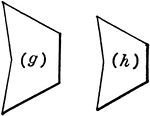### Similar Figures

2 pentagons that are similar figures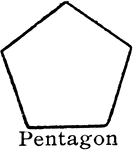### Pentagon

"A polygon of five sides." -—Hallock 1905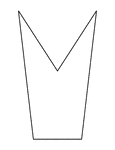### Irregular Concave Pentagon

Illustration of an irregular pentagon. This is also an example of a concave polygon with symmetry.### Irregular Concave Pentagon

Illustration of an irregular concave pentagon.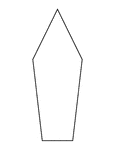### Irregular Convex Pentagon

Illustration of an irregular pentagon. This is also an example of a convex polygon with symmetry.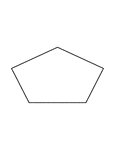### Irregular Convex Pentagon

Illustration of an irregular pentagon. This is also an example of a convex polygon with symmetry.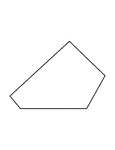### Irregular Convex Pentagon

Illustration of an irregular convex pentagon.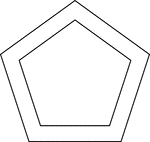### 2 Concentric Pentagons

Illustration of 2 concentric pentagons.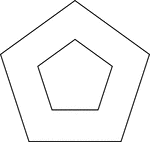### 2 Concentric Pentagons

Illustration of 2 concentric pentagons.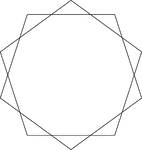### 2 Congruent Rotated Pentagons

Illustration of 2 regular congruent pentagons that have the same center. One pentagon has been rotated…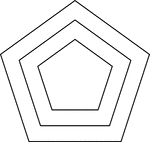### 3 Concentric Pentagons

Illustration of 3 concentric pentagons that are equally spaced.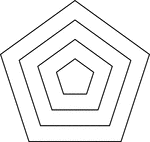### 4 Concentric Pentagons

Illustration of 4 concentric pentagons that are equally spaced.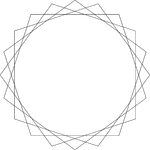### 4 Congruent Rotated Pentagons

Illustration of 4 regular congruent pentagons that have the same center. Each pentagon has been rotated…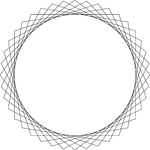### 8 Congruent Rotated Pentagons

Illustration of 8 regular congruent pentagons that have the same center. Each pentagon has been rotated…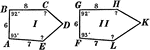### Congruent Pentagons

Illustration showing two congruent pentagons.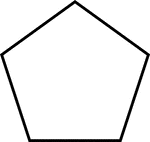### 5-sided Polygon

Polygon consisting of 5 sides### Convex Polygon

Illustration of a convex polygon. A polygon is convex when no side when produced will enter the polygon.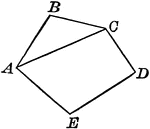### Polygon With Diagonal

Illustration of a polygon with a diagonal drawn. A diagonal of a polygon is a line joining the vertices…### Exterior Angles of Polygons

Illustration of a polygon with exterior angles drawn.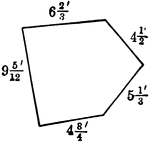### Pentagon With Sides Labeled

Illustration of a pentagon with sides measuring 6 2/3', 4 1/2', 5 1/3', 4 3/4', and 9 5/12'.